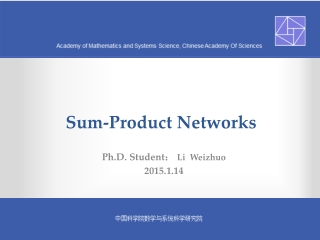DownloadDownload PresentationSum-Product Networks

Sum-Product Networks

Download PresentationSum-Product Networks

- - - - - - - - - - - - - - - - - - - - - - - - - - - E N D - - - - - - - - - - - - - - - - - - - - - - - - - - -
Presentation Transcript

1. Sum-Product Networks Ph.D. Student： Li Weizhuo 2015.1.14

2. Outline • Motivation • Representation • Inference • Learning

3. Motivation • Graphical Models

4. Motivation • Learning Graphical Model

5. Outline • Motivation • Representation • Inference • Learning

6. Representation • What does an SPN mean? • How to use SPNs to represent other networks? • The Context Specific independence(CSI)

7. What Does an SPN mean?

8. A Univariate Distribution is a SPN

9. A Product of SPNs over a Disjoint Variables is an SPN

10. A Weighted Sum of SPNs over the Same variables is an SPN

11. How to use SPNs to represent other networks? • BN  SPN • MNSPN • Mixture Model  SPN

12. BN →SPN ? ? ?

13. BN →SPN

14. BN →SPN

15. BN →SPN ? ? ? ? ?

16. MN →SPN

17. The Context Specific Independence(CSI)

18. An example in Ontology Matching ? ? ? ? ? ? • SPN  (Sims  Map| Disjoint1) • SPN  (Sims  Map| Disjoint0)

19. An example in Ontology Matching (Cont) • Context-specific independence • SPN(Map(Y1,Y2)Similarities(Y1,Y2))|Disjointwith(Y1,Y2)1) X1 Z2 SubClassof Map Disjoint Y2 Y1 X2

20. Outline • Motivation • Representation • Inference • Learning

21. Inference • All marginals are computable in time linear in size of SPN. • All MAP states are computable in time linear in size of SPN.

22. Compute marginals ? ? ? ? ? P(X=0)=? 0.74 0.5 0.9 1 0.9 0.5 1 0 1 1 1 1

23. Compute MAP ? ? ? 0.12 ? Max 0.3 0.04 0.6 0.1 0.5 0.4 Max Max Max ? Max 1 0 1 1 1

24. Outline • Motivation • Representation • Inference • Learning

25. Learning • Generative weight learning • Discriminative weight learning • Structure Learning

26. Generative weight learning(Poon,H & Domingos, UAI (2011))

27. Generative weight learning(Poon,H & Domingos, UAI (2011)) Random forest Hard EM

28. Discriminative weight learning(Gens,R & Domingos, NIPS(2012)) Bottom-Up

29. Structure Learning(Gens,R & Domingos, ICML(2013)) Mutual information Hard EM

30. Summary Maybe Nothing!

31. Summary

32. References • Most of the materials come from Domingo's slides. • Source code • http://spn.cs.washington.edu/code.shtml • video • http://videolectures.net/nips2012_gens_discriminative_learning/ • http://research.microsoft.com/apps/video/default.aspx?id=192562&r=1

33. Thanks! Q&A